Predicting and hyperparameters tuning
Support Vector Machines (SVMs) with Scikit-learn

## Support Vector Machines (SVMs)¶

Introduction

• Support vector machines are models that learn to differentiate between data in two categories based on past examples
• We want to have the maximum margin from the line to the points as shown in the diagram and that is the essence of SVMs
• The points close to the decision boundary matters, the rest are not important
• Note that SVMs aim to classify correctly before maximizing the margin
•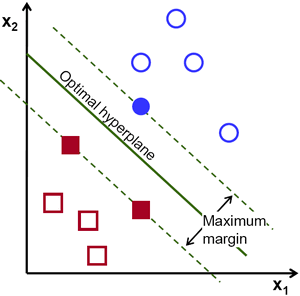• You can also project your data into a higher dimensionality and split them with a hyperplane
• Optimization problem for finding maximum margins uses quadratic programming
• We use a kernel to identify similarity amongst points
• All kernels must satisfy Mercer conditions

SVM using Scikit-Learn

In :
from sklearn.svm import SVC
from sklearn.cross_validation import train_test_split
from sklearn.metrics import accuracy_score
import seaborn as sns

In :
# Create object

# Create data
X = iris.data
y = iris.target

# Split data
X_train, X_test, y_train, y_test = train_test_split(X, y, random_state=0)

In :
# Same 3 steps

# 1. Instantiate
# Default kernel='rbf'
# We can change to others
svm = SVC(kernel='linear')

# 2. Fit
svm.fit(X_train, y_train)

# 3. Predict
y_pred = svm.predict(X_test)

In :
# Accuracy calculation
acc = accuracy_score(y_pred, y_test)
acc

Out:
0.97368421052631582

How about data that do not seem linearly separable?

• We add a non-linear feature makes SVMs capable of linearly separating the data
• As you can see here, it seems that we cannot draw a linear line to split the data
•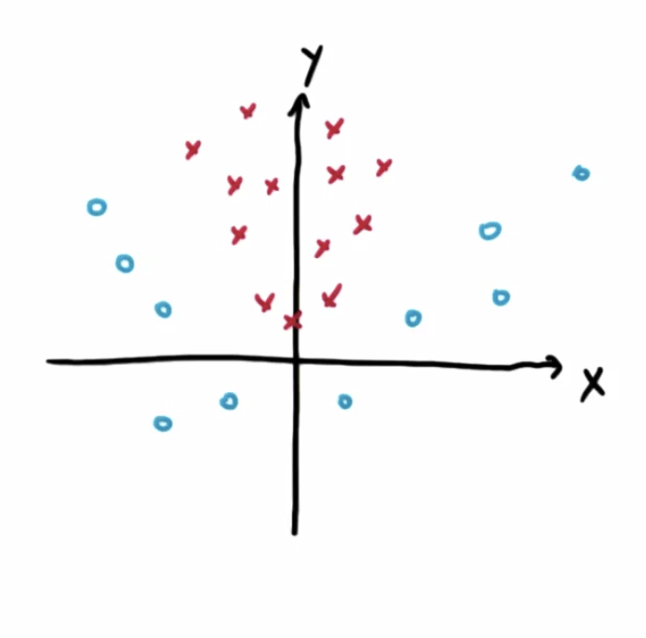• However, we can add a new feature |x| to split the data
• The new points will be linearly separable
•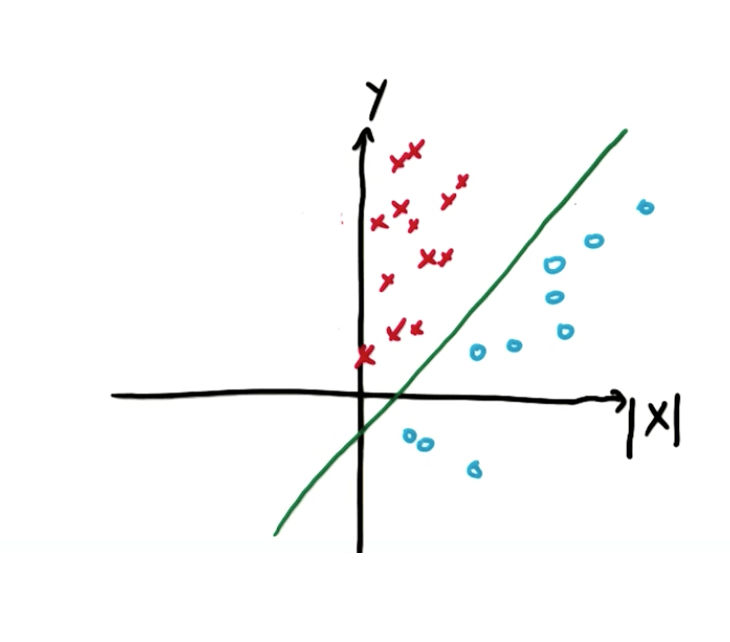• On the original plot, we can see this is how the data is separated
•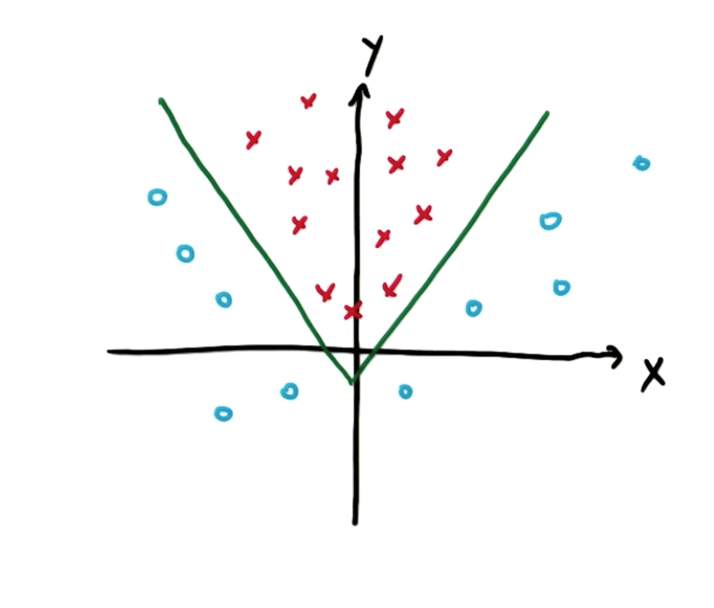• It seems like we need to create new features, but there's a cool trick called the "kernel trick"

Kernel Trick

• We can map non-separable data to a higher dimensionality to make it separable and then map back
•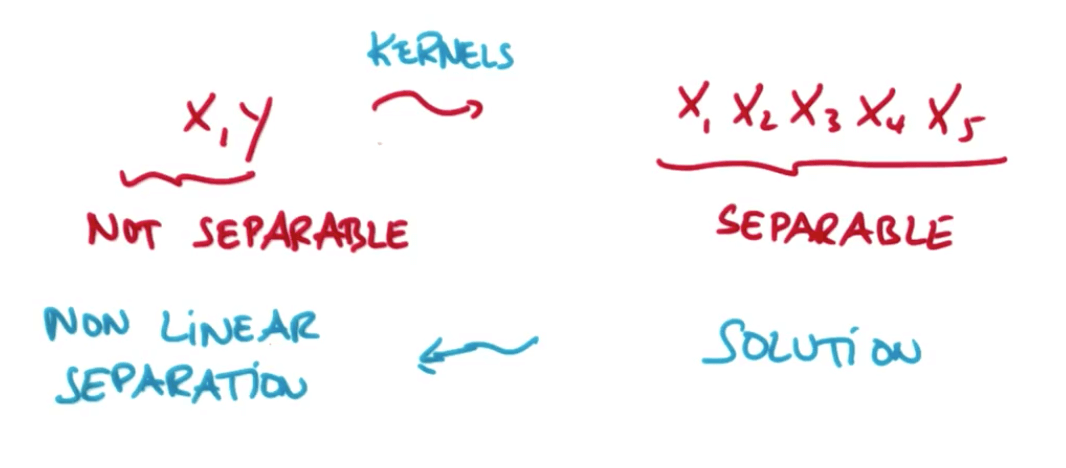Hyperparameters Tuning with Scikit-Learn

• Kernels
• You can use common kernels and custom kernels
• Common kernels
• 'linear', 'poly', 'rbf', 'sigmoid', 'precomputed'
• C
• Controls tradeoff between smooth decision boundary and classifying training points correctly
• The C parameter tells the SVM optimization how much you want to avoid misclassifying each training example
• Large C
• The optimizer will choose a smaller-margin hyperplane if that hyperplane does a better job of getting all the training points classified correctly
• Small C
• The optimizer will look for a larger-margin separating hyperplane, even if that hyperplane misclassifies more points
• For very tiny values of C, you should get misclassified examples, often even if your training data is linearly separable
• gamma
• Defines how far the influence of a single example reaches
• Low values
• Far
• Only consider close points
• High values
• Low
• Even far-away points get considered
• You can end up with a wiggly decision boundary (over-fitting)
• Kernel coefficient for ‘rbf’, ‘poly’ and ‘sigmoid’. If gamma is ‘auto’ then 1/n_features will be used instead.

SVMs Suitability

• Does not work well with large datasets due to speed issues
• Does not work well with a lot of noise
• Naive Bayes would be better
• Works well for data that can be linearly classified
Tags: